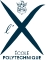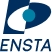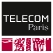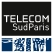Share

# Master Year 1 Applied Mathematics and StatisticsYear Master Year 1 Program Applied Mathematics and Statistics ECTS Credits 60 Language English Orientation Research or Industry Location Palaiseau Campus Course duration 12 months, full time Course start September Degree awarded Master’s degree obtained on completion of a second year of Master

## WHY ENROLL IN THIS PROGRAM?

### Asset n° 1

Build your own curriculum in mathematical sciences through a wide variety of courses, seminars, projects and internships

### Asset n°2

Get ready for a career in research and benefit from close links with the Institut Polytechnique de Paris laboratories

### Asset n°3

Prepare for a PhD through PhD tracks in Mathematics for Finance or Data Science and Artificial Intelligence

START OF SCHOOL YEAR : September 4th 2023

BEGINNING OF CLASSES: September 5th 2023

The mathematical level of this master's program is probably much higher than that of other master's programs offered by other universities in the same "mention"  "Applied Mathematics and Statistics". We only recruit students with excellent mathematical skills at BsC level. It is essential that you have taken a course in probability at the level of Ross, "Introduction to Probability Models", and a course in mathematical statistics at the level of Hogg, Mc Kean and Craig 'Introduction to Mathematical Statistics'. Must have taken a solid course in mathematical analysis.

This first-year Master’s program offers a wide range of basic and more specialized courses in applied mathematics. This allow students to build a personalized curriculum adapted to their academic and professional projects in the following areas:

• Statistics, finance and actuarial science
• Modeling, probability and artificial Intelligence
• Optimization
• Signal, computing and machine learning
• Numerical analysis and EDP

Students can also follow PhD Tracks to guide them towards a doctorate at the end of the Master:

• PhD track Mathematics for Finance (link)
• PhD track Data Science and Artificial Intelligence (link)

### Objectives

This program allows students to:

• Acquire a solid foundation in applied mathematics to pursue doctoral studies or directly apply for a mathematics-related position in an academy, business or industry
• Delve deeper into applied mathematics by addressing current, open problems
• Develop a strong relationship with research through seminars, mentoring projects and internships in research labs or companies

To complete the Master’s degree, first-year students can enter the following second-year programs:

On completing the second year of the Master, graduates can apply for PhD funding in top research labs or a job requiring advanced knowledge of applied mathematics and statistics.

Core courses

5 mandatory courses [14 weeks, 4 hours a week, 6 ECTS / Course]

• Elements of functional analysis and measure theory: topology, measure and integration theory – Lebesgue measure, Lebesgue integral abstract measure spaces, classical functional spaces – Banach, Hilbert, bounded and unbounded operators, weak topologies
• Probability theory and stochastic process :  Conditional expectations, discrete-time stochastic processes [Kolmogorov extension theorem, canonical processes, stopping times], martingales [convergence theorems, main stopping theorems, applications], continuous state-space Markov chains [transition kernels, main convergence theorems for atomic chains]
• Mathematical Statistics : introduction to decision theory, estimators (M- and Z- estimators, sufficiency, optimality), tests (constructions, Uniformly Most Powerful test for simple and composite alternatives), asymptotic statistics (consistency, asymptotic normality), asymptotic optimality of maximum likelihood estimation, generalized likelihood ratio tests, introduction to linear and non-linear regression, Bayesian statistics.
• Optimization: Optimization in Rn (general case and convex case), Optimization under equality and inequality constraints, KKT, convex case, Farkas lemma, duality, Dynamic programming techniques: discrete-time dynamic programming (finite-horizon problems; infinite-horizon problems with discounted cost), Introduction to optimal control theory (Pontriaguine principle, Hamilton-Jacobi-Bellman equation).
• Python for Data Sciences: Python is a high-level programming language, which is primarily used in thefields of data science and artificial intelligence, among others. Its design iscentered around readability, which lowers learning barriers for newcomers.
The objective of this course is to introduce Python in the context of data sciences.

3 mandatory courses :

• Markov Processes and applications [6 ECTS] : Poisson process, jump process, Markov property, simulations of Markov processes, martingales, Kolmogorov equations, ergodic theorem, long time behavior, Brownian motion

• Introduction to Machine Learning [6 ECTS] Introduction to Supervised Learning, Linear Models, Generalizes Linear Models, Support Vector Machines, Generative Learning (Gaussian discriminant analysis, Mixture models, Naïve Bayes model), Tree-based and Ensemble Models (Decision tree, random forests, boosting, bagging), learning theory (Union bound, Hoeffding inequality, Empirical risk, Probably approximately correct framework), Introduction to Unsupervised Learning (Gaussian Mixture Model, Expectation-Maximization, k-means clustering, Hierarchical clustering), Dimensionality Reduction (Principal component analysis (Eigenvalues/vectors, Spectral theorem, PCA algorithm) ● Independent component analysis (Bell and Sejnowski ICA algorithm,  Linear discriminant analysis, Factor analysis), Neural Networks (Basic architecture: multi-layer perceptron, Activation functions -Sigmoid, tanh, relu, lrelu etc.-, Losses (cross-entropy, l1/l2 loss, binary cross entropy, focal loss, hinge-loss etc.-, Back propagation, learning rate

• Databases [6 ECTS] : ● Introduction, Data Models: Relational Model ● Relational Algebra, Relational Calculus ● SQL (Structured Query Language) ● Schema Refinement and Normal Forms ● Storage and Indexes ● Query Processing (Evaluation of Operators)Query Optimization (query plans, algebraic  ● equivalence, plan space, cost estimation)

• FLE/Languages: [3 ECTS]

2 elective courses :

The first part of this course is devoted to studying univariate time series: first we present the principal statistical concepts, then estimation methods and tests; we examine the non-stationarity problem by studying the main unit root tests from a practical angle. The course is illustrated with practical examples. The second part of the course is devoted to studying stationary VAR models: we briefly present the general framework of multivariate stationary series before developing the specific case of VAR models. Finally, we take a quick look at the principles of cointegration.

The aim of this course is to present the mathematical concepts used to model and value derivatives in finance. The presentation of the course will be mathematically rigorous, but certain results from stochastic calculus will be admitted as read, to be demonstrated later in the third-year stochastic calculus course. After obtaining a mathematical definition of the concept of arbitrage in a financial market, we will study discrete models for each evolutionary tree of assets, giving useful intuitions for the study of continuous-time models. Finally, with the help of the theory of stochastic calculus , we will present asset valuation in the framework of the Black & Scholes model. It is advisable to have taken the course on Markov chains for a better understanding of the concepts from stochastic calculus and to take the simulation course in parallel.

• Numerical Analysis I & 2 - TELECOM Paris  - 5 ECTS

https://synapses.telecom-paris.fr/catalogue/2022-2023/ue/2087/macs205a-numerical-analysis-part-i

Numerical methods come into play in applied mathematics as soon as the numerical value for some quantity of interest is needed, for which no analytic expression is available.  This quantity may be e.g. anintegral or the solution of a differential equation.
The scope of application of this course thus embraces such diverse fields as Physics, Biology, Economy or Finance.  The role played by numerical
analysis has substantially grown these past few years, together with extensive computing power.

Numerical methods may be divided into two broad classes: deterministic methods on the one hand, based on discrete approximations of integrals or state evolution equations, and stochastic methods on the other hand, which consist in random sampling under an appropriate probability distribution in order to approximate an integral or atrajectory. This may be done by independent sampling (Monte-Carlo methods) or by simulating a well chosen Markov Chain (MCMC methods).

The purpose of this course is to introduce the students to essential methods in Numerical Analysis and to give them the key tools for understanding the mathematical principles behind.

### Internship

Each M1 student must complete an internship spanning a minimum duration of 8 weeks as an integral part of their education, for which they receive 7 ECTS. This internship can be completed at a college / university, in an industrial research laboratory, or in a company. The start of the internship is scheduled for May.

This mandatory internship serves as a crucial bridge between theoretical knowledge and practical application. It not only provides students with invaluable professional insights and networking opportunities, but also helps them refine or solidify their academic and professional goals for their M2 year and beyond. A comprehensive evaluation of this internship is based on a detailed report submitted by the student and a subsequent oral defense. In addition, evaluation from the internship supervisor plays a critical role in the overall assessment

Completion of a bachelor’s degree in mathematics, mathematical sciences or related field in France or abroad.

The exercises proposed in the following link allow you to evaluate the level expected to enter our master : click here

• English

#### How to apply

Applications can be submitted exclusively online. You will need to provide the following documents:

• Transcript
• CV/resume
• Statement of purpose

You will receive an answer in your candidate space within 2 months of the closing date for the application session.

### Fees and scholarships

Fees for 2023-2024 are :

• EU/EEA/Switzerland students: 4648€
• Non-EU/EEA/Switzerland students: 6839€
• Engineer students enrolled in one of the five member schools of Institut Polytechnique de Paris (Ecole polytechnique, ENSTA Paris, ENSAE Paris, Télécom Paris and Télécom SudParis): 159€
• Special cases: please refer to the "Cost of studies" section of the FAQs

### Program Office

Nathalie Rodrigues

### General enquiries

Description

START OF SCHOOL YEAR : September 4th 2023

BEGINNING OF CLASSES: September 5th 2023

The mathematical level of this master's program is probably much higher than that of other master's programs offered by other universities in the same "mention"  "Applied Mathematics and Statistics". We only recruit students with excellent mathematical skills at BsC level. It is essential that you have taken a course in probability at the level of Ross, "Introduction to Probability Models", and a course in mathematical statistics at the level of Hogg, Mc Kean and Craig 'Introduction to Mathematical Statistics'. Must have taken a solid course in mathematical analysis.

This first-year Master’s program offers a wide range of basic and more specialized courses in applied mathematics. This allow students to build a personalized curriculum adapted to their academic and professional projects in the following areas:

• Statistics, finance and actuarial science
• Modeling, probability and artificial Intelligence
• Optimization
• Signal, computing and machine learning
• Numerical analysis and EDP

Students can also follow PhD Tracks to guide them towards a doctorate at the end of the Master:

• PhD track Mathematics for Finance (link)
• PhD track Data Science and Artificial Intelligence (link)

### Objectives

This program allows students to:

• Acquire a solid foundation in applied mathematics to pursue doctoral studies or directly apply for a mathematics-related position in an academy, business or industry
• Delve deeper into applied mathematics by addressing current, open problems
• Develop a strong relationship with research through seminars, mentoring projects and internships in research labs or companies

To complete the Master’s degree, first-year students can enter the following second-year programs:

On completing the second year of the Master, graduates can apply for PhD funding in top research labs or a job requiring advanced knowledge of applied mathematics and statistics.

Core courses

5 mandatory courses [14 weeks, 4 hours a week, 6 ECTS / Course]

• Elements of functional analysis and measure theory: topology, measure and integration theory – Lebesgue measure, Lebesgue integral abstract measure spaces, classical functional spaces – Banach, Hilbert, bounded and unbounded operators, weak topologies
• Probability theory and stochastic process :  Conditional expectations, discrete-time stochastic processes [Kolmogorov extension theorem, canonical processes, stopping times], martingales [convergence theorems, main stopping theorems, applications], continuous state-space Markov chains [transition kernels, main convergence theorems for atomic chains]
• Mathematical Statistics : introduction to decision theory, estimators (M- and Z- estimators, sufficiency, optimality), tests (constructions, Uniformly Most Powerful test for simple and composite alternatives), asymptotic statistics (consistency, asymptotic normality), asymptotic optimality of maximum likelihood estimation, generalized likelihood ratio tests, introduction to linear and non-linear regression, Bayesian statistics.
• Optimization: Optimization in Rn (general case and convex case), Optimization under equality and inequality constraints, KKT, convex case, Farkas lemma, duality, Dynamic programming techniques: discrete-time dynamic programming (finite-horizon problems; infinite-horizon problems with discounted cost), Introduction to optimal control theory (Pontriaguine principle, Hamilton-Jacobi-Bellman equation).
• Python for Data Sciences: Python is a high-level programming language, which is primarily used in thefields of data science and artificial intelligence, among others. Its design iscentered around readability, which lowers learning barriers for newcomers.
The objective of this course is to introduce Python in the context of data sciences.

3 mandatory courses :

• Markov Processes and applications [6 ECTS] : Poisson process, jump process, Markov property, simulations of Markov processes, martingales, Kolmogorov equations, ergodic theorem, long time behavior, Brownian motion

• Introduction to Machine Learning [6 ECTS] Introduction to Supervised Learning, Linear Models, Generalizes Linear Models, Support Vector Machines, Generative Learning (Gaussian discriminant analysis, Mixture models, Naïve Bayes model), Tree-based and Ensemble Models (Decision tree, random forests, boosting, bagging), learning theory (Union bound, Hoeffding inequality, Empirical risk, Probably approximately correct framework), Introduction to Unsupervised Learning (Gaussian Mixture Model, Expectation-Maximization, k-means clustering, Hierarchical clustering), Dimensionality Reduction (Principal component analysis (Eigenvalues/vectors, Spectral theorem, PCA algorithm) ● Independent component analysis (Bell and Sejnowski ICA algorithm,  Linear discriminant analysis, Factor analysis), Neural Networks (Basic architecture: multi-layer perceptron, Activation functions -Sigmoid, tanh, relu, lrelu etc.-, Losses (cross-entropy, l1/l2 loss, binary cross entropy, focal loss, hinge-loss etc.-, Back propagation, learning rate

• Databases [6 ECTS] : ● Introduction, Data Models: Relational Model ● Relational Algebra, Relational Calculus ● SQL (Structured Query Language) ● Schema Refinement and Normal Forms ● Storage and Indexes ● Query Processing (Evaluation of Operators)Query Optimization (query plans, algebraic  ● equivalence, plan space, cost estimation)

• FLE/Languages: [3 ECTS]

2 elective courses :

The first part of this course is devoted to studying univariate time series: first we present the principal statistical concepts, then estimation methods and tests; we examine the non-stationarity problem by studying the main unit root tests from a practical angle. The course is illustrated with practical examples. The second part of the course is devoted to studying stationary VAR models: we briefly present the general framework of multivariate stationary series before developing the specific case of VAR models. Finally, we take a quick look at the principles of cointegration.

The aim of this course is to present the mathematical concepts used to model and value derivatives in finance. The presentation of the course will be mathematically rigorous, but certain results from stochastic calculus will be admitted as read, to be demonstrated later in the third-year stochastic calculus course. After obtaining a mathematical definition of the concept of arbitrage in a financial market, we will study discrete models for each evolutionary tree of assets, giving useful intuitions for the study of continuous-time models. Finally, with the help of the theory of stochastic calculus , we will present asset valuation in the framework of the Black & Scholes model. It is advisable to have taken the course on Markov chains for a better understanding of the concepts from stochastic calculus and to take the simulation course in parallel.

• Numerical Analysis I & 2 - TELECOM Paris  - 5 ECTS

https://synapses.telecom-paris.fr/catalogue/2022-2023/ue/2087/macs205a-numerical-analysis-part-i

Numerical methods come into play in applied mathematics as soon as the numerical value for some quantity of interest is needed, for which no analytic expression is available.  This quantity may be e.g. anintegral or the solution of a differential equation.
The scope of application of this course thus embraces such diverse fields as Physics, Biology, Economy or Finance.  The role played by numerical
analysis has substantially grown these past few years, together with extensive computing power.

Numerical methods may be divided into two broad classes: deterministic methods on the one hand, based on discrete approximations of integrals or state evolution equations, and stochastic methods on the other hand, which consist in random sampling under an appropriate probability distribution in order to approximate an integral or atrajectory. This may be done by independent sampling (Monte-Carlo methods) or by simulating a well chosen Markov Chain (MCMC methods).

The purpose of this course is to introduce the students to essential methods in Numerical Analysis and to give them the key tools for understanding the mathematical principles behind.

### Internship

Each M1 student must complete an internship spanning a minimum duration of 8 weeks as an integral part of their education, for which they receive 7 ECTS. This internship can be completed at a college / university, in an industrial research laboratory, or in a company. The start of the internship is scheduled for May.

This mandatory internship serves as a crucial bridge between theoretical knowledge and practical application. It not only provides students with invaluable professional insights and networking opportunities, but also helps them refine or solidify their academic and professional goals for their M2 year and beyond. A comprehensive evaluation of this internship is based on a detailed report submitted by the student and a subsequent oral defense. In addition, evaluation from the internship supervisor plays a critical role in the overall assessment

Completion of a bachelor’s degree in mathematics, mathematical sciences or related field in France or abroad.

The exercises proposed in the following link allow you to evaluate the level expected to enter our master : click here

• English

#### How to apply

Applications can be submitted exclusively online. You will need to provide the following documents:

• Transcript
• CV/resume
• Statement of purpose

You will receive an answer in your candidate space within 2 months of the closing date for the application session.

### Fees and scholarships

Fees for 2023-2024 are :

• EU/EEA/Switzerland students: 4648€
• Non-EU/EEA/Switzerland students: 6839€
• Engineer students enrolled in one of the five member schools of Institut Polytechnique de Paris (Ecole polytechnique, ENSTA Paris, ENSAE Paris, Télécom Paris and Télécom SudParis): 159€
• Special cases: please refer to the "Cost of studies" section of the FAQs